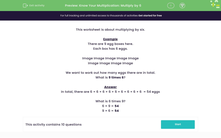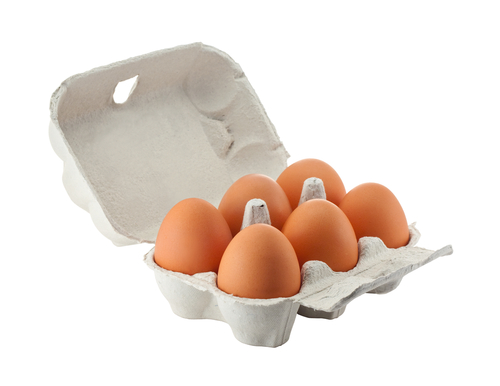# Practise Your Six Times Table

In this worksheet, students will learn to multiply by 6.Key stage:  KS 2

Curriculum topic:   Number: Multiplication and Division

Curriculum subtopic:   Know Multiplication Tables up to 12 x 12

Popular topics:   Multiplication worksheets

Difficulty level:#### Worksheet Overview

This activity is about multiplying by six.

Example

There are 9 egg boxes here.

Each box has 6 eggs.We want to work out how many eggs there are in total.

What is 9 times 6?

In total, there are 6 + 6 + 6 + 6 + 6 + 6 + 6 + 6 + 6  = 54 eggs

What is 6 times 9?

6 × 9 = 54

9 × 6 = 54

Can you see how much quicker it is to use multiplication, rather than addition?Let's have a go at the questions and practise those times tables!

### What is EdPlace?

We're your National Curriculum aligned online education content provider helping each child succeed in English, maths and science from year 1 to GCSE. With an EdPlace account you’ll be able to track and measure progress, helping each child achieve their best. We build confidence and attainment by personalising each child’s learning at a level that suits them.

Get started